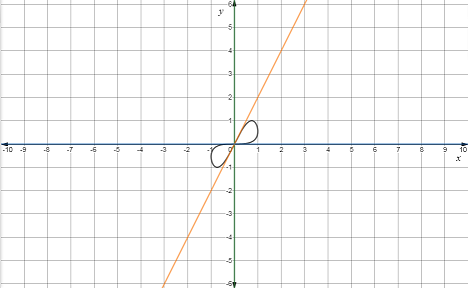# Explain that the given curve has two tangent lines at the origin.### Single Variable Calculus: Concepts...

4th Edition
James Stewart
Publisher: Cengage Learning
ISBN: 9781337687805### Single Variable Calculus: Concepts...

4th Edition
James Stewart
Publisher: Cengage Learning
ISBN: 9781337687805

#### Solutions

Chapter 3.4, Problem 84E
To determine

## Explain that the given curve has two tangent lines at the origin.

Expert Solution

### Explanation of Solution

Given:

The given equations are x=sint and y=sin(t+sint) .

Calculation:

Find the slope.

Apply formula dydx=dydtdxdt .

dydt=ddt[sin(t+sint)]

Apply chain rule.

Let f=sina,a=t+sint

dydt=dda(sina)ddt(t+sint)

Apply sum/difference rule.

(f±g)'=f'±g'

dydt=dda(sina)[ddt(t)+ddt(sint)]

Use derivative rule ddx(xn)=nxn1 and ddx(sinx)=cosx .

dydt=cosa[1+cost]

Substitute the value of a=t+sint .

dydt=cos(t+sint)[1+cost]

dxdt=sint

Use derivative rules ddx(sinx)=cosx

dxdt=cost

dydx(slope)=cos(t+sint)[1+cost]cost

Find the value of the parameter t at the origin.

sint=0t=[0,π]

At t=0 .

dydx(slope)=cos(t+sint)[1+cost]cost=cos(0+sin(0))[1+cos(0)]cos(0)=cos(0)[1+1]1=121=2

At t=π .

dydx(slope)=cos(t+sint)[1+cost]cost=cos(π+sin(π))[1+cos(π)]cos(π)=cos(π+0)1=0

There are two different tangents at the origin because there is two different slope values.

Now use point-slope form for the tangent equations.

yy1=m(xx1)y1=0,x1=0,m=2y0=2(x0)y=2xandy1=0,x1=0,m=0y0=0(x0)y=0

The graph of the given curve and the tangent lines is given below.### Have a homework question?

Subscribe to bartleby learn! Ask subject matter experts 30 homework questions each month. Plus, you’ll have access to millions of step-by-step textbook answers!